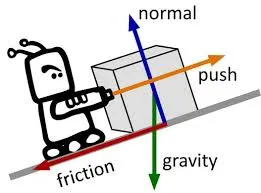# Physics - Classical mechanics - Force and Newton's first lawHello everybody it's a me again drifter1! Today we continue with Physics and more specifically Classical mechanics by starting another topic. We already covered the different types of motions in my previous posts by also getting into some examples/exercises, and this means that from now we will cover Newton's motion laws. Today's we post will be about Forces and the first law. So, without futher do, let's get started!

## Why study Forces?

Force is applied to objects so that they go out of rest and start moving. Depending on the type of Force, the angle, position etc. that Force is applied at and also the meter/value of that Force, an object moves in another way that can be analyzed using Physics. So, using Newton's Laws we will be able to calculate the Force needed to push an object so that it reaches a specific velocity or a specific point/position at a specific time and so on...

## What is Force?

Force is how we describe the interaction between 2 or more objects and the surroundings.

Because we have an direction and value, Force is a vector quantity,

Force is calculated in Newton's (N), where 1N equals the Force of an 1kg object.

The funny thing is that an apple almost weights 1kg. And as you might know already an apple fell to Newton's head and then he started writing his theory, but I think that this is made up and is not true :PYou can find some typical Force values here in wikipedia.

The same way as we added the other Vectors we can also add Force vectors. This also means that we can "split" a Force vector into it's component vectors for each axis and the sum of those will be the Force we "splitted".

Any Force can be replaced by her components that are applied at the same point!The Superposition principle in Classical Mechanics tells us that if two or more Forces are applied at the same point, then the Force applied is the vector addition of the other Forces and so:

R = F1 + F2 + ... = ΣF (sum of F's)

ΣF is the so called Net or total Force and we can calculate it for each axis independently like that:

Rx = F1x + F2x + ... = ΣFx (sum of Fx's)

Ry = F1y + F2y + ... = ΣFy (sum of Fy's)

The meter/value of ΣF=R is:

R = root(Rx^2 + Ry^2)

The direction is tanθ = Ry / Rx

And we can also apply on 3 axes with Rz = ΣFz and so:

R = root(Rx^2 + Ry^2 + Rz^2)

So, let's now get into Newton's First Law!

## Newton's First Law

The first law tells us exactly that:This means that an object tends to keep it's motion state, which can be:

• staying at rest
• staying in an linear constant velocity motion

It only changes it's state if it is forced to by a Force

(see what I did there? xD).

So, if no Force is applied or the total Force applied is zero the object moves with constant linear velocity (which can also be zero at rest) and has zero acceleration.

The tendency of staying at rest or moving with a constant velocity is called inertia and represents the laziness of objects!

So, the next time someone tells you why you are lazy just tell him: "My inertia is really high today" :P

To solve Physics problems that need the First Law (balancing) we use the following:

R = F1 + F2 + ... = ΣF = 0

which only applies if the components of R are also zero and so:

ΣFx = 0

ΣFy = 0

I will not get into Examples, cause it's actually self-explanatory. We will get into Examples/Exercises for all of the Laws when we are finished with them!

### Image Sources:

https://encrypted-tbn0.gstatic.com/images?q=tbn:ANd9GcSZPDl42G9V-MsDnTBgQlwFb7T3CxOv1Kl1cDDT94S1_schx_X6And this is actually it for today and I hope that you enjoyed it!
Next time we will get into Newton's second law and Mass!

Bye!

H2
H3
H4
3 columns
2 columns
1 column